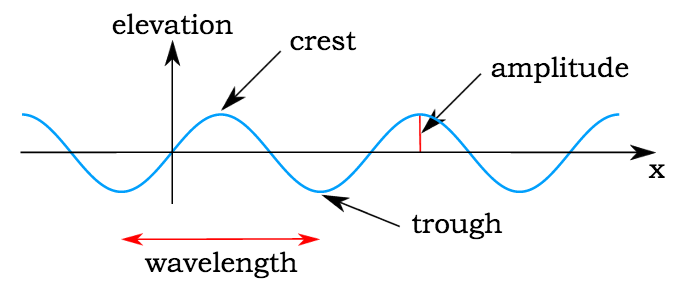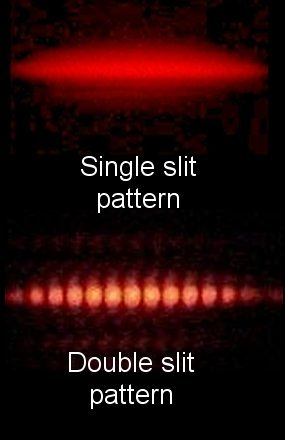## Properties of Waves and Light

#### Learning Objective

• Discuss how wave motion arises and its measurable properties, noting the conlcusions of Young’s double slit experiment

#### Key Points

• Wave motion arises when a periodic disturbance of some kind is propagated through an elastic medium. Pressure variations through air, transverse motions along a guitar string, or variations in the intensities of the local electric and magnetic fields in space, known as electromagnetic radiation, are all examples of waves.
• There are three measurable properties of wave motion: amplitude, wavelength, and frequency.
• A definitive experiment was Young’s double slit experiment, which demonstrated that light shined at two slits in a screen show an interference pattern characteristic of waves of light, rather than particles.
• The phase associated with a wave is also important in describing certain phenomena.
• The velocity of a wave is the product of the wavelength and the frequency.

#### Terms

• amplitudeThe maximum value of the variable reached in either direction.
• waveA shape that alternatively varies between a maximum in two opposite directions.
• frequencyThe number of vibrations per second.
• wavelengthThe distance traveled by the wave in a full period (1/frequency).

In this section, we will focus on the wave-like properties of light. While you will later learn about wave/particle duality (how light behaves as both a wave and a particle at the same time), here we shall discuss the wave nature of light and the experimental effects of this behavior.

## Introduction to Wave Motion

Wave motion arises when a periodic disturbance of some kind is propagated through a medium. Pressure variations through air, transverse motions along a guitar string, or variations in the intensities of the local electric and magnetic fields in space, which constitute electromagnetic radiation, are all typical examples of wave motion. For each medium, there is a characteristic velocity at which the disturbance travels.Sinusoidal waveThis image shows the anatomy of a sine curve: the crest is the peak of each wave, and the trough is the valley; the amplitude is the distance between the crest and the x-axis; and the wavelength is the distance between two crests (or two troughs).

There are three measurable properties of wave motion: amplitude, wavelength, and frequency (the number of vibrations per second). The relation between the wavelength λ (Greek lambda) and frequency of a wave ν (Greek nu) is determined by the propagation velocity v, such that

$v=\nu \lambda$

For light, this equation becomes

$\nu = \frac{c}{\lambda}$

where c is the speed of light, 2.998 x 108 m/s.

When utilizing these equations to determine wavelength, frequency, or velocity by manipulation of the equation, it is important to note that wavelengths are expressed in units of length, such as meters, centimeters, nanometers, etc; and frequency is typically expressed as megahertz or hertz (s–1).

## Example

What is the wavelength of the musical note A = 440 hz when it is propagated through air in which the velocity of sound is 343 m s–1?

λ = v (343 m s-1)/ v(440 s–1) = 0.780 m

## Young’s Double-Slit Experiment

In the early 19th century, English scientist Thomas Young carried out the famous double-slit experiment (also known as Young’s experiment), which demonstrated that a beam of light, when split into two beams and then recombined, will show interference effects that can only be explained by assuming that light is a wavelike disturbance. If light consisted strictly of ordinary or classical particles, and these particles were fired in a straight line through a slit and allowed to strike a screen on the other side, we would expect to see a pattern corresponding to the size and shape of the slit. However, when this single-slit experiment is actually performed, the pattern on the screen is a diffraction pattern in which the light is spread out. The smaller the slit, the greater the angle of spread.Young’s double slit experimentIf light were purely a particle, it would not exhibit the interference pattern shown here.

Similarly, if light consisted strictly of classical particles and we illuminated two parallel slits, the expected pattern on the screen would simply be the sum of the two single-slit patterns. In actuality, however, the pattern changes to one with a series of alternating light and dark bands. When Thomas Young first demonstrated this phenomenon, it indicated that light consists of waves, as the distribution of brightness can be explained by the alternately additive and subtractive interference of wavefronts. Young’s experiment, performed in the early 1800’s, played a vital part in the acceptance of the wave theory of light, superseding the corpuscular theory of light proposed by Isaac Newton, which had been the accepted model of light propagation in the 17th and 18th centuries. Almost a century later, in 1905, Albert Einstein’s Nobel-Prize winning research into the photoelectric effect demonstrated that light can behave as if it is composed of discrete particles under certain conditions. These seemingly contradictory discoveries made it necessary to go beyond classical physics and take the quantum nature of light into account.